Elementary Algebra

# 2.5Solve Equations with Fractions or Decimals

Elementary Algebra2.5 Solve Equations with Fractions or Decimals

### Learning Objectives

By the end of this section, you will be able to:

• Solve equations with fraction coefficients
• Solve equations with decimal coefficients

### Be Prepared 2.5

Before you get started, take this readiness quiz.

1. Multiply: $8·38.8·38.$
If you missed this problem, review Example 1.69.
2. Find the LCD of $5656$ and $1414$.
If you missed this problem, review Example 1.82.
3. Multiply 4.78 by 100.
If you missed this problem, review Example 1.98.

### Solve Equations with Fraction Coefficients

Let’s use the general strategy for solving linear equations introduced earlier to solve the equation, $18x+12=1418x+12=14$.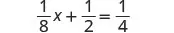To isolate the $xx$ term, subtract $1212$ from both sides.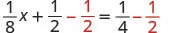Simplify the left side.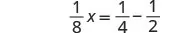Change the constants to equivalent fractions with the LCD.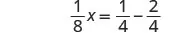Subtract.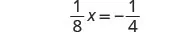Multiply both sides by the reciprocal of $1818$.Simplify.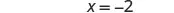This method worked fine, but many students do not feel very confident when they see all those fractions. So, we are going to show an alternate method to solve equations with fractions. This alternate method eliminates the fractions.

We will apply the Multiplication Property of Equality and multiply both sides of an equation by the least common denominator of all the fractions in the equation. The result of this operation will be a new equation, equivalent to the first, but without fractions. This process is called “clearing” the equation of fractions.

Let’s solve a similar equation, but this time use the method that eliminates the fractions.

### Example 2.48

#### How to Solve Equations with Fraction Coefficients

Solve: $16y−13=5616y−13=56$.

### Try It 2.95

Solve: $14x+12=5814x+12=58$.

### Try It 2.96

Solve: $18x+12=1418x+12=14$.

Notice in Example 2.48, once we cleared the equation of fractions, the equation was like those we solved earlier in this chapter. We changed the problem to one we already knew how to solve! We then used the General Strategy for Solving Linear Equations.

### How To

#### Strategy to solve equations with fraction coefficients.

1. Step 1. Find the least common denominator of all the fractions in the equation.
2. Step 2. Multiply both sides of the equation by that LCD. This clears the fractions.
3. Step 3. Solve using the General Strategy for Solving Linear Equations.

### Example 2.49

Solve: $6=12v+25v−34v6=12v+25v−34v$.

### Try It 2.97

Solve: $7=12x+34x−23x7=12x+34x−23x$.

### Try It 2.98

Solve: $−1=12u+14u−23u−1=12u+14u−23u$.

In the next example, we again have variables on both sides of the equation.

### Example 2.50

Solve: $a+34=38a−12a+34=38a−12$.

### Try It 2.99

Solve: $x+13=16x−12x+13=16x−12$.

### Try It 2.100

Solve: $c+34=12c−14c+34=12c−14$.

In the next example, we start by using the Distributive Property. This step clears the fractions right away.

### Example 2.51

Solve: $−5=14(8x+4)−5=14(8x+4)$.

### Try It 2.101

Solve: $−11=12(6p+2)−11=12(6p+2)$.

### Try It 2.102

Solve: $8=13(9q+6)8=13(9q+6)$.

In the next example, even after distributing, we still have fractions to clear.

### Example 2.52

Solve: $12(y−5)=14(y−1)12(y−5)=14(y−1)$.

### Try It 2.103

Solve: $15(n+3)=14(n+2)15(n+3)=14(n+2)$.

### Try It 2.104

Solve: $12(m−3)=14(m−7)12(m−3)=14(m−7)$.

### Example 2.53

Solve: $5x−34=x25x−34=x2$.

### Try It 2.105

Solve: $4y−73=y64y−73=y6$.

### Try It 2.106

Solve: $−2z−54=z8−2z−54=z8$.

### Example 2.54

Solve: $a6+2=a4+3a6+2=a4+3$.

### Try It 2.107

Solve: $b10+2=b4+5b10+2=b4+5$.

### Try It 2.108

Solve: $c6+3=c3+4c6+3=c3+4$.

### Example 2.55

Solve: $4q+32+6=3q+544q+32+6=3q+54$.

### Try It 2.109

Solve: $3r+56+1=4r+333r+56+1=4r+33$.

### Try It 2.110

Solve: $2s+32+1=3s+242s+32+1=3s+24$.

### Solve Equations with Decimal Coefficients

Some equations have decimals in them. This kind of equation will occur when we solve problems dealing with money or percentages. But decimals can also be expressed as fractions. For example, $0.3=3100.3=310$ and $0.17=171000.17=17100$. So, with an equation with decimals, we can use the same method we used to clear fractions—multiply both sides of the equation by the least common denominator.

### Example 2.56

Solve: $0.06x+0.02=0.25x−1.50.06x+0.02=0.25x−1.5$.

### Try It 2.111

Solve: $0.14h+0.12=0.35h−2.40.14h+0.12=0.35h−2.4$.

### Try It 2.112

Solve: $0.65k−0.1=0.4k−0.350.65k−0.1=0.4k−0.35$.

The next example uses an equation that is typical of the money applications in the next chapter. Notice that we distribute the decimal before we clear all the decimals.

### Example 2.57

Solve: $0.25x+0.05(x+3)=2.850.25x+0.05(x+3)=2.85$.

### Try It 2.113

Solve: $0.25n+0.05(n+5)=2.950.25n+0.05(n+5)=2.95$.

### Try It 2.114

Solve: $0.10d+0.05(d−5)=2.150.10d+0.05(d−5)=2.15$.

### Section 2.5 Exercises

#### Practice Makes Perfect

Solve Equations with Fraction Coefficients

In the following exercises, solve each equation with fraction coefficients.

318.

$1 4 x − 1 2 = − 3 4 1 4 x − 1 2 = − 3 4$

319.

$3 4 x − 1 2 = 1 4 3 4 x − 1 2 = 1 4$

320.

$5 6 y − 2 3 = − 3 2 5 6 y − 2 3 = − 3 2$

321.

$5 6 y − 1 3 = − 7 6 5 6 y − 1 3 = − 7 6$

322.

$1 2 a + 3 8 = 3 4 1 2 a + 3 8 = 3 4$

323.

$5 8 b + 1 2 = − 3 4 5 8 b + 1 2 = − 3 4$

324.

$2 = 1 3 x − 1 2 x + 2 3 x 2 = 1 3 x − 1 2 x + 2 3 x$

325.

$2 = 3 5 x − 1 3 x + 2 5 x 2 = 3 5 x − 1 3 x + 2 5 x$

326.

$1 4 m − 4 5 m + 1 2 m = −1 1 4 m − 4 5 m + 1 2 m = −1$

327.

$5 6 n − 1 4 n − 1 2 n = −2 5 6 n − 1 4 n − 1 2 n = −2$

328.

$x + 1 2 = 2 3 x − 1 2 x + 1 2 = 2 3 x − 1 2$

329.

$x + 3 4 = 1 2 x − 5 4 x + 3 4 = 1 2 x − 5 4$

330.

$1 3 w + 5 4 = w − 1 4 1 3 w + 5 4 = w − 1 4$

331.

$3 2 z + 1 3 = z − 2 3 3 2 z + 1 3 = z − 2 3$

332.

$1 2 x − 1 4 = 1 12 x + 1 6 1 2 x − 1 4 = 1 12 x + 1 6$

333.

$1 2 a − 1 4 = 1 6 a + 1 12 1 2 a − 1 4 = 1 6 a + 1 12$

334.

$1 3 b + 1 5 = 2 5 b − 3 5 1 3 b + 1 5 = 2 5 b − 3 5$

335.

$1 3 x + 2 5 = 1 5 x − 2 5 1 3 x + 2 5 = 1 5 x − 2 5$

336.

$1 = 1 6 ( 12 x − 6 ) 1 = 1 6 ( 12 x − 6 )$

337.

$1 = 1 5 ( 15 x − 10 ) 1 = 1 5 ( 15 x − 10 )$

338.

$1 4 ( p − 7 ) = 1 3 ( p + 5 ) 1 4 ( p − 7 ) = 1 3 ( p + 5 )$

339.

$1 5 ( q + 3 ) = 1 2 ( q − 3 ) 1 5 ( q + 3 ) = 1 2 ( q − 3 )$

340.

$1 2 ( x + 4 ) = 3 4 1 2 ( x + 4 ) = 3 4$

341.

$1 3 ( x + 5 ) = 5 6 1 3 ( x + 5 ) = 5 6$

342.

$5 q − 8 5 = 2 q 10 5 q − 8 5 = 2 q 10$

343.

$4 m + 2 6 = m 3 4 m + 2 6 = m 3$

344.

$4 n + 8 4 = n 3 4 n + 8 4 = n 3$

345.

$3 p + 6 3 = p 2 3 p + 6 3 = p 2$

346.

$u 3 − 4 = u 2 − 3 u 3 − 4 = u 2 − 3$

347.

$v 10 + 1 = v 4 − 2 v 10 + 1 = v 4 − 2$

348.

$c 15 + 1 = c 10 − 1 c 15 + 1 = c 10 − 1$

349.

$d 6 + 3 = d 8 + 2 d 6 + 3 = d 8 + 2$

350.

$3 x + 4 2 + 1 = 5 x + 10 8 3 x + 4 2 + 1 = 5 x + 10 8$

351.

$10 y − 2 3 + 3 = 10 y + 1 9 10 y − 2 3 + 3 = 10 y + 1 9$

352.

$7 u − 1 4 − 1 = 4 u + 8 5 7 u − 1 4 − 1 = 4 u + 8 5$

353.

$3 v − 6 2 + 5 = 11 v − 4 5 3 v − 6 2 + 5 = 11 v − 4 5$

Solve Equations with Decimal Coefficients

In the following exercises, solve each equation with decimal coefficients.

354.

$0.6 y + 3 = 9 0.6 y + 3 = 9$

355.

$0.4 y − 4 = 2 0.4 y − 4 = 2$

356.

$3.6 j − 2 = 5.2 3.6 j − 2 = 5.2$

357.

$2.1 k + 3 = 7.2 2.1 k + 3 = 7.2$

358.

$0.4 x + 0.6 = 0.5 x − 1.2 0.4 x + 0.6 = 0.5 x − 1.2$

359.

$0.7 x + 0.4 = 0.6 x + 2.4 0.7 x + 0.4 = 0.6 x + 2.4$

360.

$0.23 x + 1.47 = 0.37 x − 1.05 0.23 x + 1.47 = 0.37 x − 1.05$

361.

$0.48 x + 1.56 = 0.58 x − 0.64 0.48 x + 1.56 = 0.58 x − 0.64$

362.

$0.9 x − 1.25 = 0.75 x + 1.75 0.9 x − 1.25 = 0.75 x + 1.75$

363.

$1.2 x − 0.91 = 0.8 x + 2.29 1.2 x − 0.91 = 0.8 x + 2.29$

364.

$0.05 n + 0.10 ( n + 8 ) = 2.15 0.05 n + 0.10 ( n + 8 ) = 2.15$

365.

$0.05 n + 0.10 ( n + 7 ) = 3.55 0.05 n + 0.10 ( n + 7 ) = 3.55$

366.

$0.10 d + 0.25 ( d + 5 ) = 4.05 0.10 d + 0.25 ( d + 5 ) = 4.05$

367.

$0.10 d + 0.25 ( d + 7 ) = 5.25 0.10 d + 0.25 ( d + 7 ) = 5.25$

368.

$0.05 ( q − 5 ) + 0.25 q = 3.05 0.05 ( q − 5 ) + 0.25 q = 3.05$

369.

$0.05 ( q − 8 ) + 0.25 q = 4.10 0.05 ( q − 8 ) + 0.25 q = 4.10$

#### Everyday Math

370.

Coins Taylor has $2.00 in dimes and pennies. The number of pennies is 2 more than the number of dimes. Solve the equation $0.10d+0.01(d+2)=20.10d+0.01(d+2)=2$ for $dd$, the number of dimes. 371. Stamps Paula bought$22.82 worth of 49-cent stamps and 21-cent stamps. The number of 21-cent stamps was 8 less than the number of 49-cent stamps. Solve the equation $0.49s+0.21(s−8)=22.820.49s+0.21(s−8)=22.82$ for s, to find the number of 49-cent stamps Paula bought.

#### Writing Exercises

372.

Explain how you find the least common denominator of $3838$, $1616$, and $2323$.

373.

If an equation has several fractions, how does multiplying both sides by the LCD make it easier to solve?

374.

If an equation has fractions only on one side, why do you have to multiply both sides of the equation by the LCD?

375.

In the equation $0.35x+2.1=3.850.35x+2.1=3.85$ what is the LCD? How do you know?

#### Self Check

After completing the exercises, use this checklist to evaluate your mastery of the objectives of this section.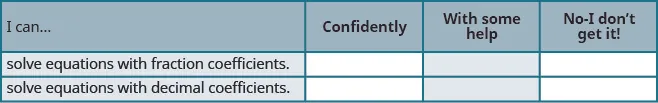Overall, after looking at the checklist, do you think you are well-prepared for the next section? Why or why not?

Order a print copy

As an Amazon Associate we earn from qualifying purchases.